# Predictability

This tutorial provides the work flow in BGGM for computing predictability in Gaussian graphical models. I will use data from a resilience questionnaire.

There are two options for computing predictability. The first simply assesses the error from the predicted values for each posterior sample. This results in a distribution of predictive error. I refer to this as fitted predictability as it is computed from the fitted values. The additional method, described below, is more Bayesian in spirit. It computes the error from the posterior predictive distribution (replicated data sets from the model).

# packages
library(BGGM)
library(ggplot2)

# resilence data
# remove gender variable
dat <- subset(rsa, select = - gender)

Note that most of the data sets in BGGM include categorical variables such as gender. Thus it is important to check the documentation to ensure that only the relevant variables are included in the analysis.

## Fitted Predictability

### Estimate the Network

The first step is to estimate the network. This provides the necessary ingredients for computing network predictability.

# fit model
fit <- estimate(dat, iter = 1000)

### Compute Predictions

Next the object fit is used to compute Bayesian variance explained.

# predict
pred <- fitted(fit, summary = FALSE)

Note summary = FALSE which returns the predicted samples for each posterior iteration. This is necessary to compute predictability.

### Compute Predictability

The next step is to compute predictability. There are several options available. In this case, I compute mean squared error.

error <- mse(pred)

# print summary
error
#> BGGM: Bayesian Gaussian Graphical Models
#> ---
#> Metric: mse
#> Type: fitted.estimate
#> Credible Interval: 0.95
#> ---
#> Estimates:
#>
#>  Node Post.mean Post.sd Cred.lb Cred.ub
#>     1      0.77    0.01    0.75    0.78
#>     2      0.83    0.01    0.81    0.85
#>     3      0.66    0.01    0.65    0.68
#>     4      0.59    0.01    0.58    0.61
#>     5      0.68    0.01    0.67    0.70
#>     6      0.75    0.01    0.74    0.77
#>     7      0.55    0.01    0.54    0.56
#>     8      0.73    0.01    0.71    0.75
#>     9      0.60    0.01    0.59    0.61
#>    10      0.78    0.01    0.77    0.80
#>    11      0.66    0.01    0.64    0.67
#>    12      0.82    0.01    0.80    0.84
#>    13      0.52    0.01    0.51    0.54
#>    14      0.86    0.01    0.84    0.88
#>    15      0.54    0.01    0.53    0.55
#>    16      0.69    0.01    0.67    0.70
#>    17      0.67    0.01    0.65    0.68
#>    18      0.47    0.01    0.46    0.48
#>    19      0.63    0.01    0.62    0.65
#>    20      0.82    0.01    0.80    0.84
#>    21      0.48    0.01    0.47    0.49
#>    22      0.77    0.01    0.76    0.80
#>    23      0.78    0.01    0.76    0.80
#>    24      0.63    0.01    0.61    0.64
#>    25      0.73    0.01    0.72    0.75
#>    26      0.71    0.01    0.69    0.72
#>    27      0.74    0.01    0.73    0.76
#>    28      0.78    0.01    0.76    0.80
#>    29      0.63    0.01    0.62    0.65
#>    30      0.86    0.01    0.84    0.88
#>    31      0.67    0.01    0.66    0.69
#>    32      0.60    0.01    0.59    0.61
#>    33      0.86    0.01    0.85    0.88

Note that the node numbers correspond to the column number in the data frame. Hence node 1 is the first column, etc.

### Plotting Predictability

#### Error Bar Plot

Most functions in BGGM have plots associated with them. In most cases, this has been simplified by calling plot.

# plot
plot(error)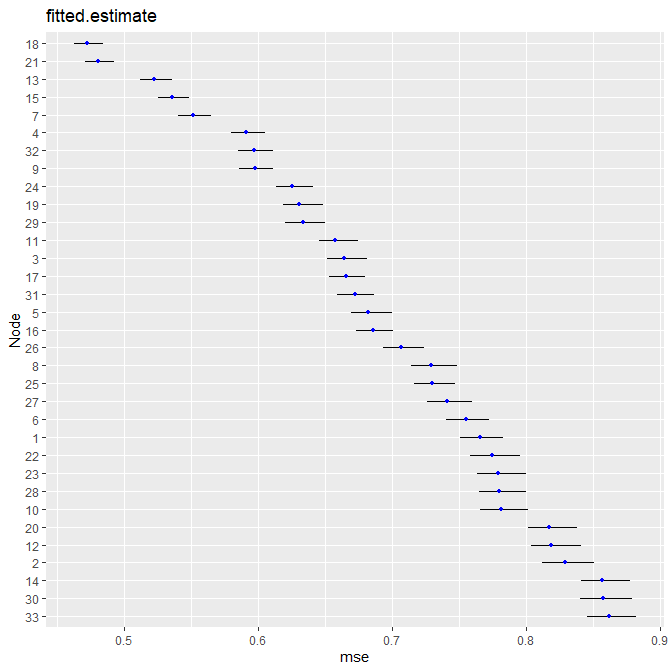This is not the most attractive plot, which is by design. The returned object is a ggplot which can then be further customized.

plot(error) +
theme_bw() +
ggtitle("Predictability") +
ylab("Mean Squared Error") +
geom_point(size = 2,
color = "black") +
geom_point(size = 1.5,
color = "white")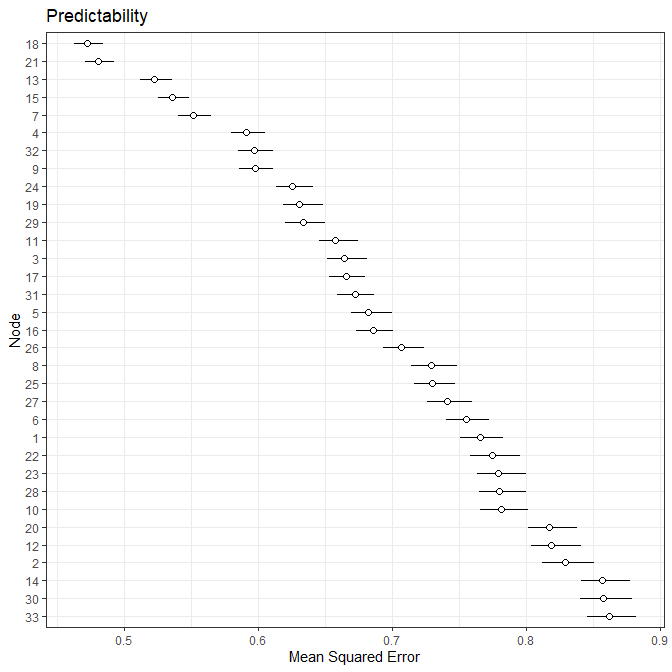#### Ridgeline Plot

It is also possible to visualize predictability with ridgelines plots.

fitted_pred <- plot(error, type = "ridgeline",
color = "red",
alpha =  0.75,
scale = 2) +
theme_bw() +
theme(legend.position = "none") +
ylab("Node") +
xlab("Mean Squared Error") +
ggtitle("Predictability")
fitted_pred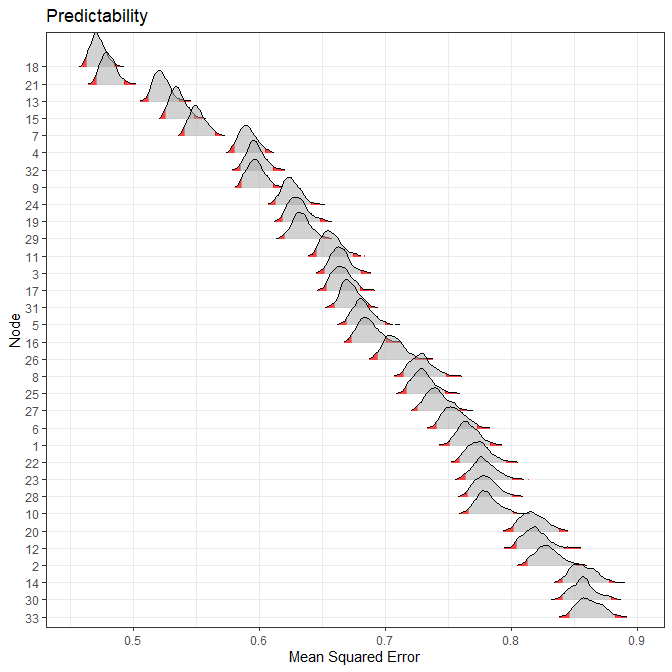## Posterior Predictive Predictability

This is implemeted with the same functions as fitted predictability. The only difference how the prediction are computed.

### Compute Predictions

pred <- posterior_predict(fit, iter = 250,
summary = FALSE)

### Compute Predictability

The next step is to compute predictability. There are several options available. In this case, I compute mean squared error.

error <- mse(pred)

### Plotting Predictability

#### Error Bar Plot

Most functions in BGGM have plots associated with them. In most cases, this has been simplified by calling plot.

# plot
plot(error)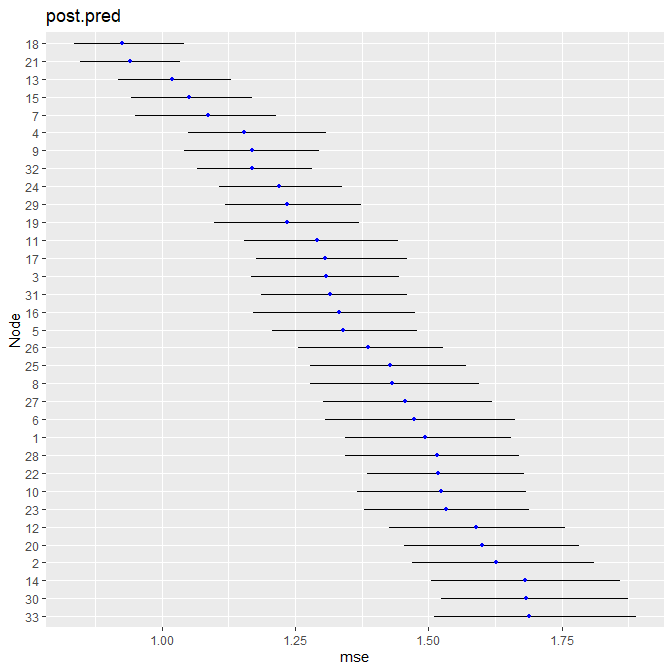This is not the most attractive plot, which is by design. The returned object is a ggplot which can then be further customized.

#### Ridgeline Plot

It is also possible to visualize predictability with ridgelines plots.

posterior_pred <- plot(error, type = "ridgeline",
color = "red",
alpha =  0.75,
scale = 2) +
theme_bw() +
theme(legend.position = "none") +
ylab("Node") +
xlab("Mean Squared Error") +
ggtitle("Predictability")
posterior_pred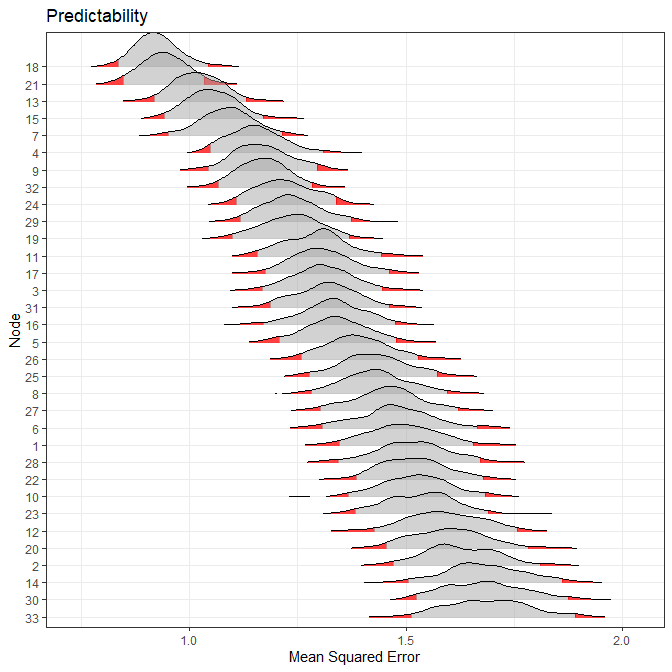## Fitted vs. Posterior Predictive Predictability

Note that the posterior predictive method account for uncertainty in the distribution of future data. Hence, the error will not only be larger but there will be more uncertainty, for example,

top <- cowplot::plot_grid("", "",
labels = c("Fitted",
"Posterior Predictive"))

bottom <- cowplot::plot_grid(fitted_pred,
posterior_pred)

cowplot::plot_grid(top, bottom,
nrow = 2,
rel_heights = c(1, 20))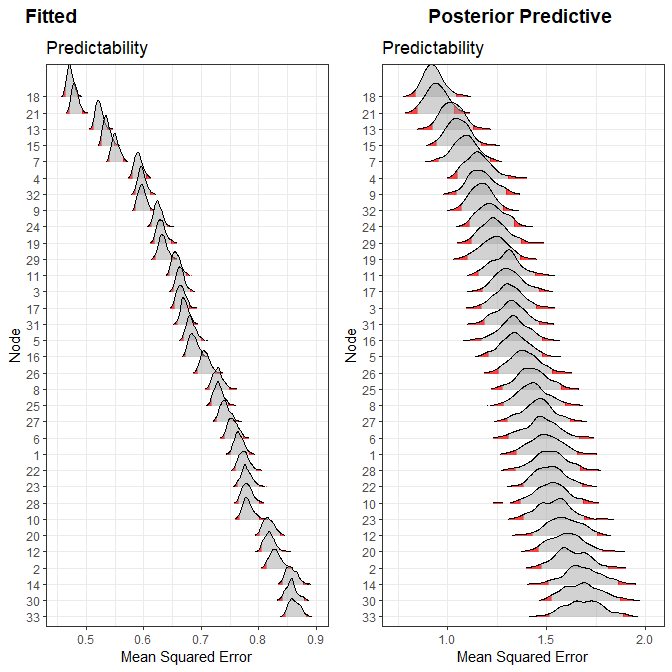## Alternative Metrics

Currently there are five metrics implemented in BGGM: (1) mean squared error (mse); (2) root mean squared error (rmse); (3) mean absolute error (mae); (4) mean absolute percentage error (mape)

The fifth metric is Bayesian variance explained. This is the focus of another vignette. And there will be a paper introudcing methodology that allows for comparing Bayesian $$R^2$$ within and between networks.